Next: Rigid body rotation Up: Lagrangian mechanics Previous: Generalized momenta

# Exercises

1. A horizontal rod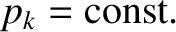rotates with constant angular velocity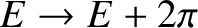about its mid-point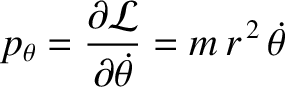. A particle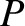is attached to it by equal-length strings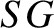,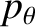. If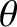is the inclination of the plane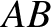to the vertical, prove that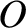where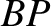. Deduce the condition that the vertical position of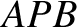should be stable.

2. A double pendulum consists of two simple pendula, with one pendulum suspended from the bob of the other. Suppose that the two pendula have equal lengths,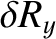, and bobs of equal mass,, and are confined to move in the same vertical plane. Letand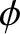--the angles that the upper and lower pendula make with the downward vertical (respectively)--be the generalized coordinates. Demonstrate that Lagrange's equations of motion for the system are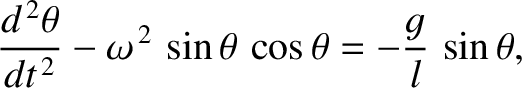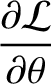and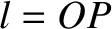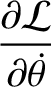3. Consider an elastic pendulum consisting of a bob of massattached to a light elastic string of stiffness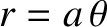and unstreatched length. Letbe the extension of the string, andthe angle that the string makes with the downward vertical. Assume that any motion is confined to a vertical plane. Demonstrate that Lagrange's equations of motion for the system are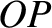and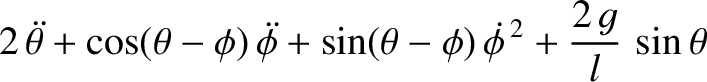4. A disk of mass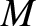and radius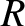rolls without slipping down a plane inclined at an angleto the horizontal. The disk has a short weightless axle of negligible radius. From this axle is suspended a simple pendulum of length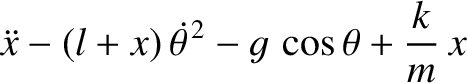whose bob is of mass. Assume that the motion of the pendulum takes place in the plane of the disk. Let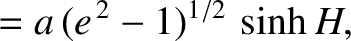be the displacement of the center of mass of the disk down the slope, and letbe the angle subtended between the pendulum and the downward vertical. Demonstrate that Lagrange's equations of motion for the system are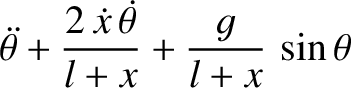and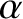=1.75in

Chapter06/fig6.01.eps

5. A vertical circular hoop of radiusis rotated in a vertical plane about a pointon its circumference at the constant angular velocity. A bead of massslides without friction on the hoop. Let the generalized coordinate be the angleshown in the diagram. Here,is a horizontal Cartesian coordinate,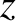a vertical Cartesian coordinate, and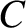the center of the hoop. Demonstrate that the equation of motion of the system is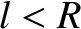(Modified from Fowles and Cassiday 2005.)

6. The kinetic energy of a rotating rigid object with an axis of symmetry can be written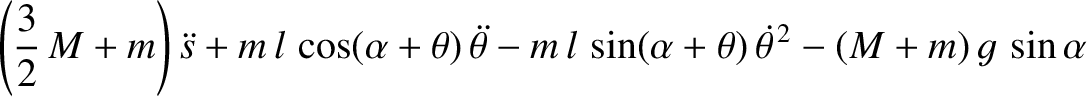where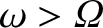is the moment of inertia about the symmetry axis,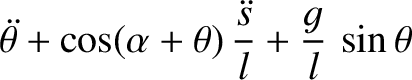is the moment of inertia about an axis perpendicular to the symmetry axis, and,,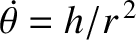are the three Euler angles. (See Chapter 8.) Suppose that the object is rotating freely. Find the momenta conjugate to the Euler angles. Which of these momenta are conserved? Find Lagrange's equations of motion for the system. Demonstrate that if the system is precessing steadily (which implies that,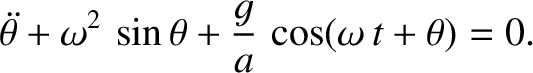, and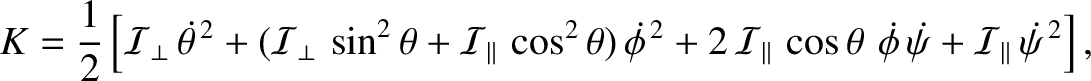are constants) then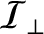7. Demonstrate that the components of acceleration in the spherical coordinate system are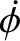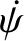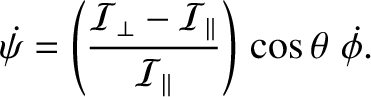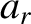and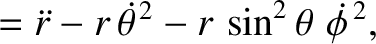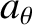(From Lamb 1923.)

8. A particle is constrained to move on a smooth spherical surface of radius. Suppose that the particle is projected with velocity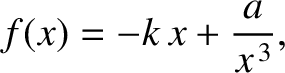along the horizontal great circle. Demonstrate that the particle subsequently falls a vertical height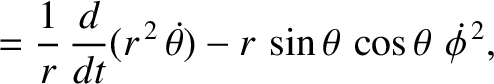, where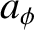Show that ifis large compared to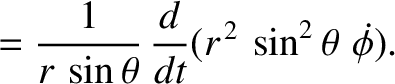then this height becomes approximately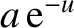. (From Lamb 1923.)

9. Consider a nonconservative system in which the dissipative forces take the form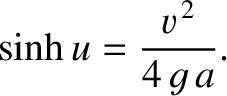, where the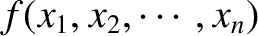are Cartesian coordinates, and the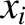are all positive. Demonstrate that the dissipative forces can be incorporated into the Lagrangian formalism provided that Lagrange's equations of motion are modified to read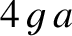where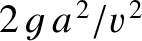is termed the Rayleigh dissipation function.Next: Rigid body rotation Up: Lagrangian mechanics Previous: Generalized momenta
Richard Fitzpatrick 2016-03-31